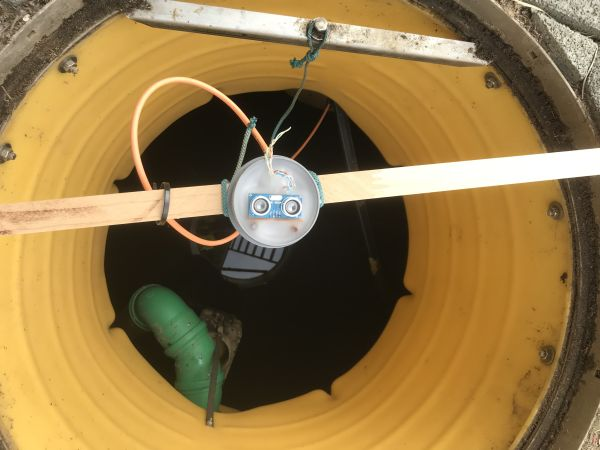Dr. Arne JachensDr. Arne Jachens

# Ultra Sonic Distance MeasurementR1 = 330 Ohm, R2 = 470 Ohm

To monitor the water storage in my cistern I decided for a HCSR04 sensor module.To avoid uncontrolled reflexes by the volume and the build in technique like pipes, I installed the sensor centrally of the manhole of the water reservoir at a height, that it is safely over the maximal gauge.## Python interface

Following this tutorial, I created my own interface to read the water level:

```import  numpy as  np
import  datetime
import  time
import  RPi.GPIO as  GPIO

class HvcHCSR04ultrasonic:
def  __init__(self):
self.GPIO_Trig = 15
self.GPIO_Echo = 14
self.speed = 34300 # cm/s
self.depth = 199   # cm
self.hMax  = 170   # cm
# my reservoir:
# D=2.1m; Hmax=1.7m; A=3.46m^2; V=5.89m^3

GPIO.setwarnings(False) #probably you already use HvcSetGPIO
GPIO.setmode(GPIO.BCM)
GPIO.setup(self.GPIO_Trig, GPIO.OUT)
GPIO.setup(self.GPIO_Echo, GPIO.IN)

def  distance(self):
# set trigger HIGH
GPIO.output(self.GPIO_Trig, GPIO.HIGH)
# set trigger LOW again to initiate measurement
time.sleep(0.00001)
GPIO.output(self.GPIO_Trig, GPIO.LOW)
start = time.time()
stop = time.time()
timeOut = time.time()
failure=False
# measurement starts, once ECHO is set HIGH
while GPIO.input(self.GPIO_Echo) == 0:
start = time.time()
if start>timeOut+0.3:
failure=True
failureType="Trigger"
break
# measurement ends, when ECHO is set LOW again
while GPIO.input(self.GPIO_Echo) == 1:
stop = time.time()
if stop>start+0.3:
failure=True
failureType="Echo"
break

# compute the distance, sound travels forth and back
if failure:
print("ERROR: ultrasonic",failureType,timeOut,start,stop)
dist=200
else:
elapsedTime = stop - start
dist = (elapsedTime * self.speed) / 2.0
#print(dist)

return dist

def  filter(self,dist):
if dist<=0:
#too short, not plausible
pass
elif dist>=self.depth:
#too long, not plausible
pass
elif HvcHCSR04ultrasonic.distFlt == -1.0:
HvcHCSR04ultrasonic.distFlt = dist
else:
w=0.1
lowPass = (1.0-w)*HvcHCSR04ultrasonic.distFlt + w*dist
HvcHCSR04ultrasonic.distFlt = lowPass

return HvcHCSR04ultrasonic.distFlt

def  volume(self,dist):
#distance to height
hRel = (self.depth-dist)/self.hMax * 100.0
return hRel

#persistant variables:
HvcHCSR04ultrasonic.distFlt = -1.0

if __name__ == "__main__":
H2O = HvcHCSR04ultrasonic()
dist = H2O.distance()
distFlt = H2O.filter(dist)
fill = H2O.volume(distFlt)
print(dist,distFlt,fill)
```

If you use GPIO14 + GPIO15, then do

```sudo raspi-config > Advanced > Serial
```
to disable the serial port.I mounted the HCSR04 in a plastic cap and after the electrical connection, I sealed everything by filling the cap with candle wax to keep the humidity off the electronis. Only the tips of speaker and microphone look out of the wax.

To avoid disturbing echos from the walls, I positioned the sensor at the center of the manhole.